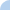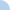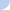# Lesson 2.2 & 2.3

Common Variable Names
Δ (delta) = the change in
δ (delta) = total deformation or elongation (in.)
σ (sigma) = stress (force per unit area, psi or lb/in.2)
ε (epsilon) = strain or unit elongation
E = modulus of elasticity
P = axial force (lb)
A = cross-sectional area (unit²)
L = length (in.)
d = diameter (in.)
p= Pressure (psi or lb/in.2)

Formulas:
Definition of normal stress: s = P/A
Definition of normal strain: e = d /L
δ = PL / AEFirst name Last nameThis activity was created by a Quia Web subscriber. Learn more about QuiaCreate your own activities